Next: Further cache coherence protocols Up: Case study Previous: Case study

## Verification of MESI cache coherence protocol

As an example we consider here the verification of the parameterised cache coherence protocol MESI. Following  it is formally modelled as a parameterised family of identical finite state automata (with four states in this case: Modified, Exclusive, Shared and Invalid) equipped with a rudimentary form of communication: when active automaton makes an action it is required that all other automata perform complementary re-action. At every step an automaton is singled out non-deterministically as an active.

Protocol encoding. We encode this protocol as a parameterised Refal program. The following fragment of Refal program describes the function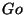which is an entry point of the program.
```\$ENTRY Go {e.A (e.I) = <Loop (e.A) (Invalid e.I)(Modified )(Shared )(Exclusive ) >;}
```
It has two input parameters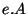and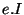which according to Refal conventions may take arbitrary Refal expressions as the values. We assume non-deterministic execution of the protocol and the characters of a string value of e.A represent a possible choices at branching points. So the length of a string value ofis the number of (non-deterministic) steps of the protocol the program is going to execute. The elements of the string value ofrepresent automata and its length is the number of automata in the system. The functiontakes two input parameters and call the function Loop (angular brackets denote a function call in Refal) with these parameters plus additional arguments describing initial condition, which in the case of MESI protocol is as follows: all automata are in the state Invalid. The function Loop is defined in as follows:
```
Loop {
() (Invalid e.1)(Modified e.2)(Shared e.3)(Exclusive e.4) =
<Result (Invalid e.1)(Modified e.2)(Shared e.3)(Exclusive e.4)>;
(s.A e.A) (Invalid e.1)(Modified e.2)(Shared e.3)(Exclusive e.4) =
<Loop (e.A)
<RandomAction s.A
(Invalid e.1)(Modified e.2)(Shared e.3)(Exclusive e.4)>>;
}
```
The definition of Loop contains two sentences: one for the quitting the loop and passing to the correctness checking and another for making recursive call of Loop with the decremented first argument value and the current state updated depending on the symbol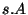. Update is done by the call to RandomAction function, which is defined as follows:
```RandomAction {

A (Invalid s.1 e.1) (Modified e.2) (Shared e.3) (Exclusive e.4)
= (Invalid e.1)  (Modified )  (Shared s.1 e.2 e.3 e.4 ) (Exclusive );

B (Invalid e.1)(Modified e.2)(Shared e.3)(Exclusive s.4 e.4) =
(Invalid e.1)(Modified s.4  e.2)(Shared e.3)(Exclusive e.4);
C (Invalid e.1)(Modified e.2)(Shared s.3 e.3)(Exclusive e.4) =
(Invalid e.4 e.3 e.2 e.1)(Modified  )(Shared )(Exclusive s.3);
D (Invalid s.1 e.1)(Modified e.2)(Shared e.3)(Exclusive e.4) =
(Invalid e.4 e.3 e.2 e.1)(Modified  )(Shared )(Exclusive s.1);
}
```
In terms of MESI parameterised finite state model symbols `A`, `B`, `C` and `D` denote four possible situations (local state of acting automaton + the action it performs) when the global state of the system is updated; all other situations, not affecting the global state, are ignored in our encoding. As an instance consider the case when RandomAction is called with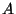as the first argument value and there is at least one automaton in the state Invalid then the state of the protocol will be updated by moving one automaton from the state Invalid, and all automata from the states Modified and Exclusive to the state Shared (cf. the first sentence of the definition).

Correctness conditions. Finally, the definition of the function Result embodies the correctness conditions of the protocol:
• in any run of the protocol at no moment two automata are in the states Modified and Shared, respectively; and
• at no moment two automata are in the state Modified.
```Result{
(Invalid e.1)(Modified s.2 e.2)(Shared s.3 e.3)(Exclusive e.4) = False;
(Invalid e.1)(Modified s.21 s.22 e.2)(Shared e.3)(Exclusive e.4) = False;

(Invalid e.1)(Modified e.2)(Shared e.3)(Exclusive e.4) = True;
}
```
Taking all together. Taking all above fragments together we get a Refal program which models the execution of the MESI protocol for the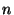steps and then checks the correctness condition on the obtained state of the protocol. The program has two parameters: the list of automata(string of symbols `e.I`) and the list of non-deterministic choices (string of symbols `e.A`). Here is the complete listing of the program:
```*****************  MESI protocol *************************************
*\$MST_FROM_ENTRY;
*\$STRATEGY Applicative;
*\$LENGTH 0;

\$ENTRY Go {e.A (e.I) = <Loop (e.A) (Invalid e.I)(Modified )(Shared )(Exclusive ) >;}

Loop {
() (Invalid e.1)(Modified e.2)(Shared e.3)(Exclusive e.4) =
<Result (Invalid e.1)(Modified e.2)(Shared e.3)(Exclusive e.4)>;
(s.A e.A) (Invalid e.1)(Modified e.2)(Shared e.3)(Exclusive e.4) =
<Loop (e.A)
<RandomAction s.A
(Invalid e.1)(Modified e.2)(Shared e.3)(Exclusive e.4)>>;
}

RandomAction {
* rh Trivial
* rm
A (Invalid s.1 e.1) (Modified e.2) (Shared e.3) (Exclusive e.4)
= (Invalid e.1)  (Modified )  (Shared s.1 e.2 e.3 e.4 ) (Exclusive );
* wh1 Trivial
*wh2
B (Invalid e.1)(Modified e.2)(Shared e.3)(Exclusive s.4 e.4) =
(Invalid e.1)(Modified s.4  e.2)(Shared e.3)(Exclusive e.4);
* wh3
C (Invalid e.1)(Modified e.2)(Shared s.3 e.3)(Exclusive e.4) =
(Invalid e.4 e.3 e.2 e.1)(Modified  )(Shared )(Exclusive s.3);
* wm
D (Invalid s.1 e.1)(Modified e.2)(Shared e.3)(Exclusive e.4) =
(Invalid e.4 e.3 e.2 e.1)(Modified  )(Shared )(Exclusive s.1);
}

Result{
(Invalid e.1)(Modified s.2 e.2)(Shared s.3 e.3)(Exclusive e.4) = False;
(Invalid e.1)(Modified s.21 s.22 e.2)(Shared e.3)(Exclusive e.4) = False;

(Invalid e.1)(Modified e.2)(Shared e.3)(Exclusive e.4) = True;
}
**************************************************************************
```
The symbol `*` denotes the start of comments according to the Refal syntax. If `*` is followed by `\$` (as in the first three lines of the program) then the rest of the line is considered as a directive for the supercompiler SCP4, still being ignored by Refal compiler. We will discuss the directives of SCP4 elsewhere. The comments inside the definition of RandomAction denote particular actions of the protocol encoded. We have borrowed these from the description of MESI protocol in , so an interested reader may match and compare two representations of the protocol.

Result of supercompilation. Now we apply the supercompiler SCP4 to that program. The result of supercompilation is the following program:
```/*
\$ENTRY Go {
= <Prout <Go e.1 >> ;
}
*/

* InputFormat: <Go e.41 >
\$ENTRY Go {
(e.101 )  = True ;
A e.41 (s.103 e.101 )  = <F24 (e.41 ) (e.101 ) s.103 > ;
D e.41 (s.104 e.101 )  = <F35 (e.41 ) (e.101 ) s.104 > ;
}

* InputFormat: <F35 (e.109 ) (e.110 ) s.111 e.112 >
F35 {
() (e.110 ) s.111 e.112  = True ;
(A e.109 ) (e.110 ) s.111 s.118 e.112  = <F24 (e.109 ) (e.112 e.110 ) s.118
s.111 > ;
(A e.109 ) (s.119 e.110 ) s.111  = <F24 (e.109 ) (e.110 ) s.119 s.111 >
;
(B ) (e.110 ) s.111 e.112  = True ;
(B A e.109 ) (e.110 ) s.111 s.125 e.112  = <F24 (e.109 ) (e.112 e.110 )
s.125 s.111 > ;
(B A e.109 ) (s.126 e.110 ) s.111  = <F24 (e.109 ) (e.110 ) s.126 s.111
> ;
(B D e.109 ) (e.110 ) s.111 s.127 e.112  = <F35 (e.109 ) (s.111 e.112 e.110
) s.127 > ;
(B D e.109 ) (s.128 e.110 ) s.111  = <F35 (e.109 ) (s.111 e.110 ) s.128
> ;
(D e.109 ) (e.110 ) s.111 s.120 e.112  = <F35 (e.109 ) (s.111 e.112 e.110
) s.120 > ;
(D e.109 ) (s.121 e.110 ) s.111  = <F35 (e.109 ) (s.111 e.110 ) s.121 >
;
}

* InputFormat: <F24 (e.109 ) (e.110 ) s.111 e.112 >
F24 {
() (e.110 ) s.111 e.112  = True ;
(A e.109 ) (s.114 e.110 ) s.111 e.112  = <F24 (e.109 ) (e.110 ) s.114 s.111
e.112 > ;
(C e.109 ) (e.110 ) s.111 e.112  = <F35 (e.109 ) (e.110 ) s.111 e.112 >
;
(D e.109 ) (s.115 e.110 ) s.111 e.112  = <F35 (e.109 ) (s.111 e.112 e.110
) s.115 > ;
}

****************************** The End ************************************
```
Interpretation of the result. At the first glance the resulting program does not look much simpler than the original one. However, it is now simple to check that:
the entire resulting program unlike the original one does not contain the expression False.
That means whatever values input parameters are given, the program will never produce False. Since the resulting program is equivalent to the original one on the domain of latter we conclude that original program will also never produce False, which implies the correctness of the encoded parameterised protocol.

Verficiation is completed. The whole process of supercompilation in this case has taken less than 1 second on the Sony Vaio laptop with Pentium III 1.2 GHz, 256 MB RAM, running Windows XP and supercompiler SCP4. The time of checking whether False is present in the resulting program is negligible.Next: Further cache coherence protocols Up: Case study Previous: Case study
Alexei Lisitsa 2005-07-14